# Transcendental properties of the certain mix infinite products

Eiji Miyanohara
Notes on Number Theory and Discrete Mathematics
Print ISSN 1310–5132, Online ISSN 2367–8275
Volume 29, 2023, Number 1, Pages 48–61
DOI: 10.7546/nntdm.2023.29.1.48-61

## Details

### Authors and affiliations

Eiji MiyanoharaTokyo, Japan

### Abstract

Let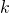andbe two multiplicatively independent positive integers and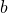be an integer with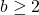. Let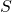be a finite set of integers. Nishioka proved that for any algebraic number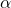with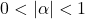the infinite products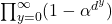(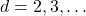) are algebraically independent over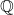. As her result, for example, the transcendence of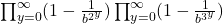is deduced. On the other hand, Tachiya, Amou–Väänänen investigated the certain infinite products which satisfy infinite chains of Mahler functional equation. The special case of the result of Tachiya shows that the infinite product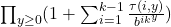with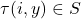(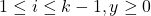) is either rational or transcendental.

In this paper, we prove that the infinite product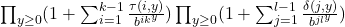with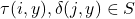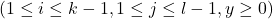is either rational or transcendental. Moreover, we give sufficient conditions thatis transcendental.

### Keywords

• Infinite product
• Transcendence
• Infinite chains of Mahler functional equations

• 11J91
• 11J87

### References

1. Allouche, J.-P., & Shallit, J. (2003). Automatic sequences: Theory, Applications,
Generalizations. Cambridge University Press.
2. Amou, M., & Väänänen, M. (2015). Arithmetical properties of certain infinite products. Journal of Number Theory, 153, 283–303.
3. Amou, M., & Väänänen, K. (2017). On algebraic independence of a class of infinite products. Journal of Number Theory, 172, 114–132.
4. Bell, J. P., Bugeaud, Y., & Coons, M. (2015). Diophantine approximation of Mahler numbers. Proceedings of the London Mathematical Society, (3) 110, 1157–1206.
5. Bugeaud, Y. (2012). Distribution modulo one and Diophantine approximation. Cambridge Tracts in Mathematics, Vol. 193. Cambridge University Press.
6. Coons, M., & Spiegelhofer, L. (2018). Number Theoretic Aspects of Regular Sequences. In V. Berthé, & M. Rigo (Eds.), Sequences, Groups, and Number Theory, Trends in Mathematics Series, Springer, pp. 37–87.
7. Delange, H. (1972). Sur les fonctions q-additives ou q-multiplicatives. Acta Arithmetica, 21, 285–298.
8. Duverney, D., & Nishioka, K. (2003). An inductive method for proving the transcendence of certain series. Acta Arithmetica, 110(4), 305–330.
9. Gel’fond, A. O. (1968). Sur les nombres qui ont des propriétés additives et multiplicatives données. Acta Arithmetica, 13, 259–265.
10. Masser, D. (2016). Auxiliary Polynomials in Number Theory. Cambridge Tracts in Mathematics Book 207.
11. Nishioka, K. (1994). Algebraic independence by Mahler’s method and S-unit equations. Compositio Mathematica, 92, 87–110.
12. Nishioka, K. (1996). Mahler Functions and Transcendence. Lecture Notes in Mathematics, Vol. 1631 Springer.
13. Tachiya, Y. (2007). Transcendence of certain infinite products. Journal of Number Theory, 125(1), 182–200.
14. Uchida, Y. (1999). On p and q-additive functions. Tokyo Journal of Mathematics, 22(1), 83–97.

### Manuscript history

• Revised: 3 January 2023
• Accepted: 15 February 2023
• Online First: 18 February 2023Ⓒ 2023 by the Author.